Size measures for items related to cells

This page lists order of magnitude estimates for the sizes of various microscopic items that are related to cells. The goal is to give a rough sense of what individual sizes mean, since these are too small for us to compare with our day-to-day intuitions.

Units for length

Units

Unit for length (full word) Unit for length (shorthand) In terms of meters (m) What's it used to measure?
femtometer fm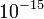$10^{-15}$ nucleus of an atom (not nucleus of a cell). Rarely used in biology
picometer pm$10^{-12}$ radius and diameter of an atom or size of a molecule. Used in biology when talking about biological membranes and passage of ions through membranes
angstrom A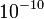$10^{-10}$ sizes of molecules, wavelengths of light
nanometer nm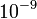$10^{-9}$ sizes of very large molecules, substructures and organelles of cells, wavelengths of light
micron or micrometer$\mu$m$10^{-6}$ sizes of cells and cellular organelles
millimeter mm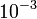$10^{-3}$ Rarely used in cell biology. May be used for tissues and cell clusters

Conversion between units

Unit for length (full word) Unit for length (shorthand) Number of fm Number of pm Number of angstroms Number of nm Number of$\mu$m Number of mm
femtometer fm 1$10^{-3}$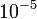$10^{-5}$$10^{-6}$$10^{-9}$$10^{-12}$
picometer pm$10^3$ 1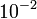$10^{-2}$$10^{-3}$$10^{-6}$$10^{-9}$
angstrom A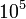$10^5$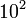$10^2$ 1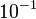$10^{-1}$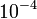$10^{-4}$$10^{-7}$
nanometer nm$10^6$$10^3$ 10 1$10^{-3}$$10^{-6}$
micron or micrometer$\mu$m$10^9$$10^6$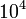$10^4$$10^3$ 1$10^{-3}$
millimeter mm$10^{12}$$10^9$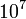$10^7$$10^6$$10^3$ 1

Lengths of items related to cell size

Item Number of meters Number of fm Number of pm Number of angstroms Number of nm Number of$\mu$m Number of mm
diameter of atomic nucleus (varies with element) = twice the nuclear radius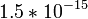$1.5 * 10^{-15}$
to$1.5 * 10^{-14}$
1.5
to
15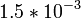$1.5 * 10^{-3}$
to$1.5 * 10^{-2}$$1.5 * 10^{-5}$
to$1.5 * 10^{-4}$$1.5 * 10^{-6}$
to$1.5 * 10^{-5}$$1.5 * 10^{-9}$
to$1.5 * 10^{-8}$$1.5 * 10^{-12}$
to$1.5 * 10^{-11}$
diameter of atom (varies with element) = twice the atomic radius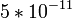$5 * 10^{-11}$
to$6 * 10^{-10}$
5000
to
60000
50
to
600
0.5
to
6
0.05
to
0.6$5 * 10^{-5}$
to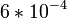$6 * 10^{-4}$$5 * 10^{-8}$
to$6 * 10^{-7}$
thickness of lipid bilayer$10^{-9}$
to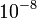$10^{-8}$$10^6$
to$10^7$
1000
to
10000
10
to
100
1
to
10$10^{-3}$
to$10^{-2}$$10^{-6}$
to$10^{-5}$
wavelength of visible light in vacuum (wavelength depends on color)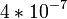$4 * 10^{-7}$
to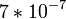$7 * 10^{-7}$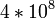$4 * 10^8$
to$7 * 10^8$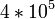$4 * 10^5$
to$7 * 10^5$
4000
to
7000
400
to
700
0.4
to
0.7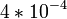$4 * 10^{-4}$
to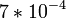$7 * 10^{-4}$
diameter of prokaryotic cell (varies based on organism)$10^{-6}$
to$10^{-5}$$10^9$
to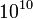$10^{10}$$10^6$
to$10^7$$10^4$
to$10^5$
1000
to
10000
1
to
10$10^{-3}$
to$10^{-2}$
diameter of eukaryotic cell (varies based on organism and cell type)$10^{-5}$
to$10^{-4}$$10^{10}$
to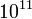$10^{11}$$10^7$
to$10^8$$10^5$
to$10^6$$10^4$
to$10^5$
10
to
100
0.01
to
0.1

Comparison of areas and volumes

The relation between ratios of lengths, areas, and volumes states that for two items with the same shape, the ratio of corresponding areas is the square of the ratio of corresponding lengths, and the ratio of corresponding volumes is the cube of the ratio of corresponding lengths. Even for objects of somewhat different shapes, the above holds to a reasonable approximation if the shapes are reasonably similar. We can use this to do quick and approximate computations of the ratio of volumes of the atom and the atomic nucleus, or the ratio of volumes of eukaryotic and prokaryotic cells.